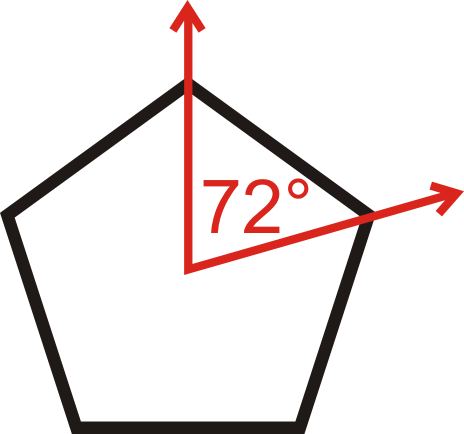# 8.4: Rotation Symmetry

•• Contributed by CK12
• CK12

Rotation less than 360 degrees that carries a shape onto itself.

Rotational symmetry is present when a figure can be rotated (less than $$360^{\circ}$$) such that it looks like it did before the rotation. The center of rotation is the point a figure is rotated around such that the rotational symmetry holds.Figure $$\PageIndex{1}$$

For the $$H$$, we can rotate it twice, the triangle can be rotated 3 times and still look the same and the hexagon can be rotated 6 times.

What if you had a six-pointed star and you rotated that star less than $$360^{\circ}$$? If the rotated star looked exactly the same as the original star, what would that say about the star?

Example $$\PageIndex{1}$$

Determine if the figure below has rotational symmetry. Find the angle and how many times it can be rotated.Figure $$\PageIndex{2}$$

Solution

The pentagon can be rotated 5 times. Because there are 5 lines of rotational symmetry, the angle would be $$\dfrac{360^{\circ}}{5}=72^{\circ}$$.Figure $$\PageIndex{3}$$Figure $$\PageIndex{4}$$

Example $$\PageIndex{2}$$

Determine if the figure below has rotational symmetry. Find the angle and how many times it can be rotated.Figure $$\PageIndex{5}$$

Solution

The N can be rotated twice. This means the angle of rotation is $$180^{\circ}$$.Figure $$\PageIndex{6}$$

Example $$\PageIndex{3}$$

Determine if the figure below has rotational symmetry. Find the angle and how many times it can be rotated.Figure $$\PageIndex{7}$$

Solution

The checkerboard can be rotated 4 times. There are 4 lines of rotational symmetry, so the angle of rotation is $$\dfrac{360^{\circ}}{4}=90^{\circ}$$.Figure $$\PageIndex{8}$$Figure $$\PageIndex{9}$$

Example $$\PageIndex{4}$$

Find the angle of rotation and the number of times each figure can rotate.Figure $$\PageIndex{10}$$

Solution

The parallelogram can be rotated twice. The angle of rotation is $$180^{\circ}$$.

Example $$\PageIndex{5}$$Figure $$\PageIndex{11}$$

Solution

The hexagon can be rotated six times. The angle of rotation is $$60^{\circ}$$.

## Review

1. If a figure has 3 lines of rotational symmetry, it can be rotated _______ times.
2. If a figure can be rotated 6 times, it has _______ lines of rotational symmetry.
3. If a figure can be rotated n times, it has _______ lines of rotational symmetry.
4. To find the angle of rotation, divide $$360^{\circ}$$ by the total number of _____________.
5. Every square has an angle of rotation of _________.

Determine whether each statement is true or false.

1. Every parallelogram has rotational symmetry.
2. Every figure that has line symmetry also has rotational symmetry.

Determine whether the words below have rotation symmetry.

1. OHIO
2. MOW
3. WOW
4. KICK
5. pod

Find the angle of rotation and the number of times each figure can rotate.

1.Figure $$\PageIndex{12}$$
2.Figure $$\PageIndex{13}$$
3.Figure $$\PageIndex{14}$$

Determine if the figures below have rotation symmetry. Identify the angle of rotation.

1.Figure $$\PageIndex{15}$$
2.Figure $$\PageIndex{16}$$
3.Figure $$\PageIndex{17}$$

## Vocabulary

Term Definition
rotational symmetry When a figure can be rotated (less than 360^{\circ}\)) such that it looks like it did before the rotation. The center of rotation is the point a figure is rotated around such that the rotational symmetry holds.
Center of Rotation In a rotation, the center of rotation is the point that does not move. The rest of the plane rotates around this fixed point.
Rotation A rotation is a transformation that turns a figure on the coordinate plane a certain number of degrees about a given point without changing the shape or size of the figure.
Rotation Symmetry A figure has rotational symmetry if it can be rotated less than $$360^{\circ}$$ around its center point and look exactly the same as it did before the rotation.
Symmetry A figure has symmetry if it can be transformed and still look the same.

Interactive Element

Video: Rotation Symmetry Principles - Basic

Activities: Rotation Symmetry Discussion Questions

Study Aids: Symmetry and Tessellations Study Guide

Practice: Rotation Symmetry

Real World: This End Up